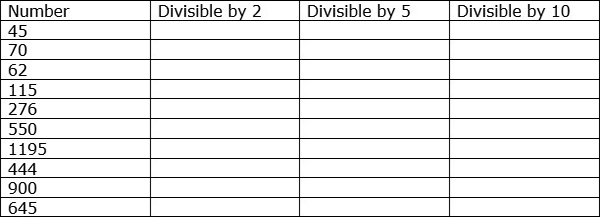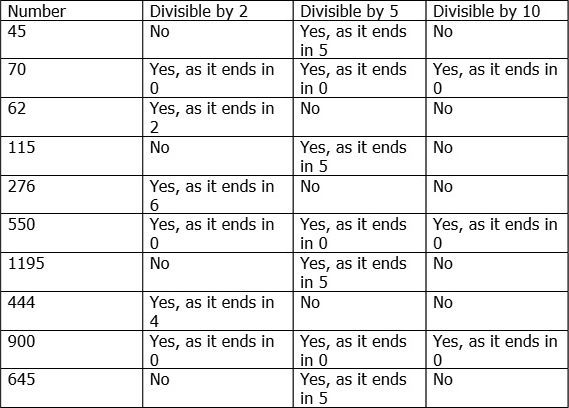# Divisibility Rules for 2, 5, and 10

Divisibility − A number m is said to be divisible by another number n

if n divides m completely without leaving a remainder.

### Divisibility Rule for 2

• If a number ends in a 0, 2, 4, 6 or 8, it is divisible by 2.
• All even numbers, by definition, are divisible by 2.

Some examples of numbers divisible by 2 are as follows.

24, 18, 68, 108, 184, 1020.

### Divisibility Rule for 5

• If a number ends in either a 0 or 5, it is divisible by 5.

Some examples of numbers divisible by 5 are

15, 35, 75, 125, 505, 1000.

### Divisibility Rule for 10

• If a number ends in a 0, it is divisible by 10.
• Also if a number is divisible by both 2 and 5, it is divisible by 10.

Some examples of numbers divisible by 10.

20, 50, 90, 110, 170, 1200.

Find the divisibility of the numbers in following table −### Solution